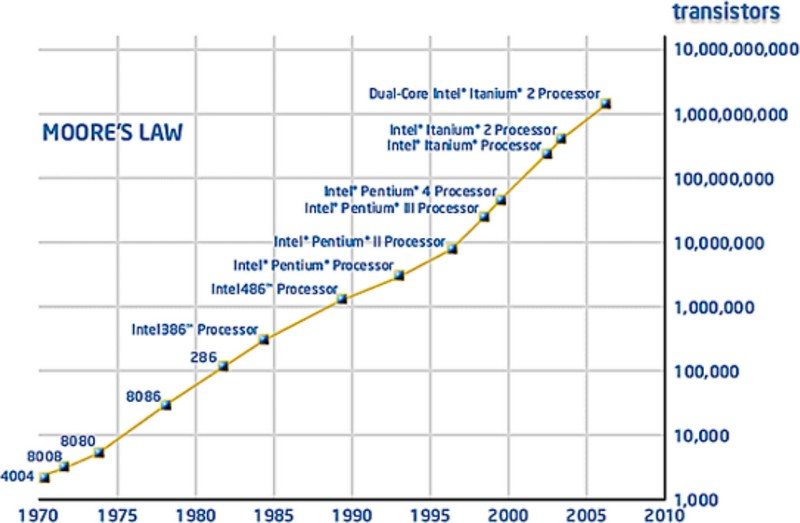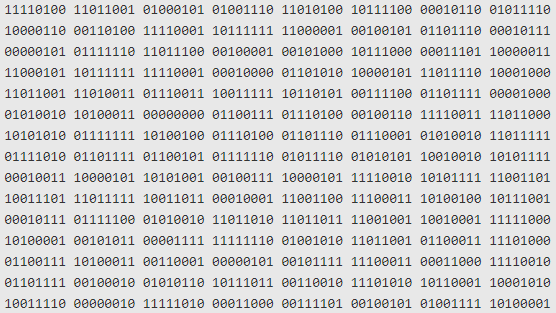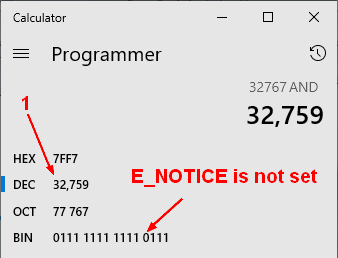# Bit - Bitwise and Shift binary operator

Operator on bit.

Bitwise binary operator may also be used as assignment operator

## Type

• the bitwise arithmetic operators (~, &, ^, and |)
• and the shift operators (<<, >>, and >>>).

### Arithmetic

The bitwise arithmetic operators

• ~ (NOT)
• & (AND)
• ^ (XOR)
• and | OR

They are also Boolean operator.

#### NOT (~)

• ~, the Bitwise NOT Operator
``````// ~x is roughly the same as -(x+1)
console.log(~2);    // -(2+1) ==> -3```
```

#### XOR (^) - Exclusive OR

The bitwise addition, modulo 2, of two bit strings of equal length.

The bitwise XOR expression follows the following rules:

``````0 ^ 0 = 0
0 ^ 1 = 1
1 ^ 0 = 1
1 ^ 1 = 0```
```

Example

````console.log(6 ^ 2); //  110 ^ 010 = 100 = 4`
```

#### OR (|)

The bitwise OR expression follows the following rules:

``````0 | 0 = 0
0 | 1 = 1
1 | 0 = 1
1 | 1 = 1```
```

Example:

``````console.log(6 | 2); //  110 ^ 010 = 110 = 6
console.log(8 | 1); //  1000 ^ 0001 = 1001 = 9```
```

#### AND (&)

``````0 & 0 = 0
0 & 1 = 0
1 & 0 = 0
1 & 1 = 1```
```

### Shift

The shift operators shifts the digits to the left or to the right:

• moving the bits to the left or to the right (if you need to position them inside a fix number of bit)
• and/or multiplying or dividing by 2

List:

• << - shift to the left (ie multiply by 2)
• >> - shift to the right (divide by 2)
• and >>>

Example / Demo:

• The initial value of 1 in bit
``````const foo = '0001'; // 1
console.log(`Initial Value: bit: \${foo}, decimal \${parseInt(foo, 2)}`);```
```
• Shift one bit to the left (multiply by 2)
``````let fooShifted = parseInt(foo, 2) << 1
console.log(`shifted by one (x2): bit: \${fooShifted.toString(2)}, decimal: \${fooShifted}`);```
```
• Shift two bit to the left (2×2)
``````fooShifted = parseInt(foo, 2) << 2
console.log(`shifted by two (x2 x2): bit: \${fooShifted.toString(2)}, decimal: \${fooShifted}`);```
```

## Documentation / Reference

Recommended PagesBit - Binary Number

Moore Law A bit also known as binary digit is the smallest unit of the binary numeral system. ie 0 or 1 byte A binary 'bit' is also known as binary digit. The term binary implies the numeral system...Boolean - (Logical) Operator (OR, AND, XOR, )

Boolean operator manipultes truthy and falsy values that can come from: a Boolean value the interpretation of a non-boolean value (ie javascript (truthy/falsy)) or the result of a comparison expression....Byte (Bit Octet) - Computer storage Unit (8bit)

(8bit) The byte is the smallest unit of computer storage and represents: 8 bits. or 2 hexadecimal (1 hexa = 4 bit) Computer storage capacity is measured in bytes. In today’s large-capacity...Computer Storage - Integer Data Type (Binary Data)

In computer, integer are stored in word from 8 to 64 bit. Because CPU manipulates integer data type, they are also sometime called binary data type. Bit Length Two's complement signed Unsigned Float...
Javascript - Number (Double)

Most programming languages have several types of numeric data, but JavaScript gets away with just one. All numbers are and are stored as doubles. See also': 32 bit 53 bit - the max of ...
Javascript - Operator (Mathematical, Comparison, ..)

in javascript SeeLanguage - Assignment

An assignment is an expression that gives a value to a variable. assignmentstatementexpression assignmentstatementassignment In its simplest form, an assignment statement has a variable on the...Language - Operator

An operator is a symbol (token) that compose an expression. It operates on values on its side (left and/or right). They represents a function but where the argument are on both side of it. This...Number - Integer -

An integer is the part of a number that is located at the left of the radix point. In other word, it's a number without its fractional component. Negative numbers without fractional components are...Php - Standard Error Handling

Php - Standard Error Handling This page is the standard error handling system of php known also as the error functions. This system: triggers error at a certain level that may be caught...